#0

## 群組

`<g>` 是 Group 的意思，其子元素會繼承該元素的屬性，常用在 `transform`

``````<svg width="250" height="250">
<g transform="scale(2, 1.3)">
<rect x="5" y="5" rx="10" ry="10" width="70" height="70" />
<rect x="100" y="5" rx="10" ry="10" width="70" height="70" />
</g>
</svg>
``````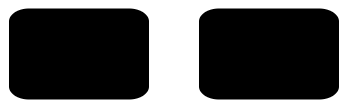## 定義

`<defs>` 是 definitions 的意思，定義在裡面的元素不會在畫面上，可以使用 `<use>` 將其繪製出來，這個標籤讓我們可以重複使用裡面的元素，使用方法需先在 `<defs>` 內定義元素，並賦予 id，然後在外側使用 `<use>`，並寫入屬性 `xlink:href="#id"`

``````<svg width="250" height="250">
<defs>
<circle id="circle" cx="0" cy="0" r="50" />
</defs>

<use x="50" y="50" xlink:href="#circle" />
</svg>
``````## 漸層

``````<svg width="250" height="250">
<defs>
<stop offset="0%" stop-color="red" stop-opacity="1" />
<stop offset="50%" stop-color="yellow" stop-opacity="1" />
<stop offset="100%" stop-color="green" stop-opacity="1" />
</defs>
<rect rx="10" ry="10" width="100" height="100" fill="url(#linearGradient)" />
</svg>
``````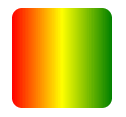• `offset`: 範圍為 0% ~ 100% 或是 0 ~ 1，兩者皆可
• `stop-color`: 漸層顏色，這邊一樣可使用 `rgba`
• `stop-opacity`: 顏色透明度

### 線性漸層

• `x1`: 第一點的 X 位置，預設值為 0
• `y1`: 第一點的 Y 位置，預設值為 0
• `x2`: 第二點的 X 位置，預設值為 1
• `y2`: 第二點的 Y 位置，預設值為 0
``````<svg width="250" height="250">
<defs>
<stop offset="0%" stop-color="red" />
<stop offset="50%" stop-color="yellow" />
<stop offset="100%" stop-color="green" />
</defs>
<rect rx="10" ry="10" width="300" height="100" fill="url(#linearGradient)" />
</svg>
``````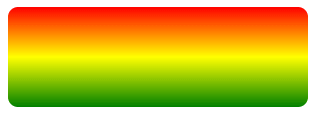### 放射性漸層

• `cx`: 漸層範圍圓心的 X 位置，預設值為 0.5
• `cy`: 漸層範圍圓心的 Y 位置，預設值為 0.5
• `r`: 半徑大小，預設值為 0.5
• `fx`: 焦點的 X 位置，預設與 cx 的值一樣
• `fy`: 焦點的 Y 位置，預設與 cy 的值一樣
• `spreadMethod`: 剩餘空間的處理方式
• `pad`: 以最外層的顏色填滿
• `repeat`: 重複漸層
• `reflect`: 這個也是重複漸層，但是他會從 0% → 100% → 0%，感覺較滑順
``````<svg width="250" height="250">
<defs>
cx="0.7" cy="0.7" r="0.7" fx="0.4" fy="0.4"
<stop offset="0%" stop-color="red" stop-opacity="1" />
<stop offset="50%" stop-color="yellow" stop-opacity="1" />
<stop offset="100%" stop-color="green" stop-opacity="1" />
</defs>
<rect rx="10" ry="10" width="300" height="100" fill="url(#radialGradient)" />
</svg>
``````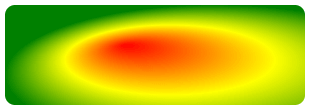### 漸層單位系統

`gradientUnits` 這個屬性是一個單位系統，部分標籤可使用此屬性，主要是切換相對單位與絕對單位

• `objectBoundingBox`: 相對單位，範圍為 0% ~ 100% 或是 0 ~ 1，此為預設值
• `userSpaceOnUse`: 絕對單位，需先知道對象位置
``````// 假設寬高皆為 100px，以下兩種方法結果相同
``````

## 樣式

`<pattern>` 一樣須在 `<defs>` 內宣告後，再將其使用於外面的元素

``````<svg width="250" height="250">
<defs>
<pattern id="pattern" width=".25" height=".25"
patternContentUnits="objectBoundingBox">
<rect x="0" y="0" width=".25" height=".25" fill="red" />
<rect x="0" y=".1" width=".25" height=".05" fill="black" />
<rect x=".1" y="0" width=".05" height=".25" fill="black" />
</pattern>
</defs>
<rect fill="url(#pattern)" width="250" height="250" />
</svg>
``````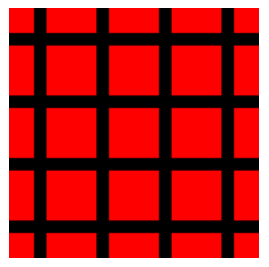### 樣式單位系統

`gradientUnits` 一樣，在樣式內也有 `patternContentUnits` 可調整單位，需要特別注意的是它的預設值為`userSpaceOnUse`，與漸層相反

## 文字

• `x`: 文字 X 軸的絕對座標
• `y`: 文字 Y 軸的絕對座標
• `dx`: 每個文字距離左側的距離，可用逗號區隔帶入每個文字的距離
• `dy`: 每個文字距離上方的距離，可用逗號區隔帶入每個文字的距離
• `text-anchor`: 設定 X 軸方向對齊方式，有 `start``middle``end` 三種
• `dominant-baseline`: 設定 Y 軸方向對齊方式，有 `baseline``middle``hanging` 三種
• `rotate`: 旋轉文字
• `textLength`: 文字的寬度，每個字的間距會自動分配，只有在絕對座標下才有作用
``````<svg width="250" height="250">
<text x="150" y="20" textLength="300"
text-anchor="middle" dominant-baseline="middle"
style="fill:red;stroke:blue;font-size:30px;">
Hello
<tspan font-weight="bold" fill="yellow" rotate="10">my</tspan>
World!
</text>
</svg>
``````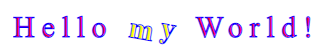### 路徑文字

``````<svg width="250" height="250">
<defs>
<path id="path" d="M20 30 Q40 5,60 30 T90 60 L200 80" />
</defs>
<text>
Hello World!
</textPath>
</text>
</svg>
``````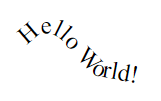## 裁切

`<defs>` 中宣告 `<clipPath>`，並在圖形中使用 `clip-path="url(#id)"` 引用，宣告的範圍會保留，其餘部份則會被移除

``````<svg width="250" height="250">
<defs>
<clipPath id="cut">
<rect x="0" y="0" width="250" height="125" />
</clipPath>
</defs>
<circle cx="125" cy="125" r="125" clip-path="url(#cut)" />
</svg>
``````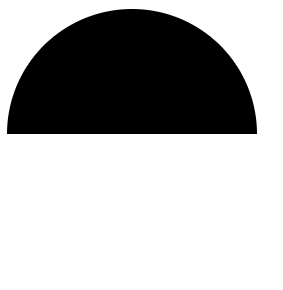## 遮蔽

`<defs>` 中宣告 `<mask>`，並一樣在圖形使用 `mask="url(#id)"` 引用，其概念同遮色片，所有顏色都會被轉為灰階，而越接近白色則越不透明，越接近黑色則越透明

``````<svg width="250" height="250">
<defs>
<stop offset="0" stop-color="black" />
<stop offset="1" stop-color="white" />
<rect x="0" y="0" width="250" height="250" fill="url(#gradient)" />
</defs>
<rect x="0" y="0" width="250" height="250"
</svg>
``````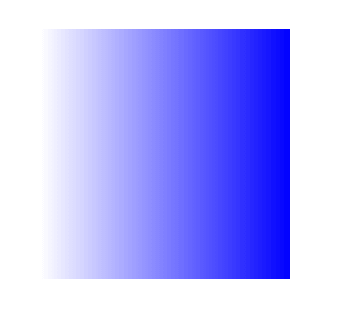## 圖片

• `x`: 圖片距離左側的距離，預設值為 0
• `y`: 圖片距離上方的距離，預設值為 0
• `width`: 圖片寬度
• `height`: 圖片高度
``````<svg width="250" height="250">
<image x="0" y="0" width="250" height="250"